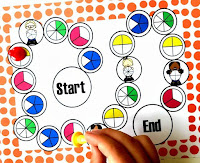# Lesson Planning of Fractions

Lesson Planning of Fractions (Proper and Improper )

Subject Mathematics

Students` Learning Outcomes

• Differentiate between proper and improper fraction

Information for Teachers

4     3      8

Material / Resources

Writing board, chalk/marker, duster, paper strips, fraction cards, fraction strips, box

Introduction

• Show different kinds of diagrams to the class.
• Ask them to tell the fraction of each diagram.
• Write the fraction on the board, as;      2,   3,   1,   5,   7,   3

4    3    2    4   5     6• Ask students how each fraction is different from other.
• Explain if students finding difficulty.
• Use proper vocabulary e.g. Proper and Improper Fraction

Development

Approach

Group work, discussion, demonstration, activities

 Activity 1 Show one paper strip to the students. Discuss how to share one strip into two students. Ask student what fraction each child has, write fraction on the board. Now take three strips and discuss how to share equally among two students. By making half of three strips each students will get three halves. Ask them to write it mathematically on the board. Make student realize that in first situation each student got less than a whole stripe but in second case each student gets more than a whole stripe. Discuss ½ and 3/2 fractions, highlight values of numerator and denominator in both cases.

 Activity 2 Write different proper and improper fractions on the paper strips. Put them in a box and give this resource to students in groups. Ask one group to collect proper fraction cards and other groups of students to collect improper fraction card. After cards collection ask each group to paste their collected cards on a chart paper, give a title ‘proper fraction’ and ‘improper fraction’. Hang chart papers in front of class and give a presentation about proper and improper fractions.

 Activity 3 Hang a fraction board (as shown in the figure) on writing board; provide a coin and a pointer to students. Ask them to flip coin, if head appears students need to hit a proper fraction with pointer from a suitable distance.If they hit correctly one point will be given to students otherwise student will lose the one mark. Each student will get three turn. Winner will be appreciated with a gift.

Sum up / Conclusion

• Sum up the lesson by asking following questions, as;
1. What is the difference between proper and improper fraction?
• Ask any two examples of proper and improper fraction.

Assessment

• Give some fractions to students and ask them to separate proper fractions and improper fraction.
• Ask students to complete the sheet by writing fraction, and also circle improper fractions.
• Teacher is also required to involve the students in solving the problems given in the exercise at the end of unit / chapter.